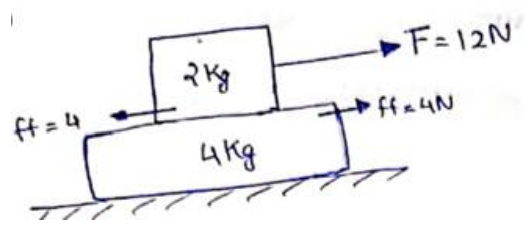Deepak Scored 45->99%ile with Bounce Back Crack Course. You can do it too!

# Solve the following :

Question:

A $2 \mathrm{~kg}$ block is placed over a $4 \mathrm{~kg}$ block and both are placed on a smooth horizontal surface. The coefficient of friction between the blocks is $0.20$. Find the acceleration of the two blocks if a horizontal force of $12 \mathrm{~N}$ is applied to (a) the upper block, (b) the lower block. Take $g=10 \mathrm{~m} / \mathrm{s}^{2}$.

Solution:

(a)Normal contact for $2 \mathrm{Kg}=2 \mathrm{~g}=20 \mathrm{~N}$.

Maximum friction force between $2 \mathrm{Kg}$ and $4 \mathrm{Kg}=\mu N$

$=02(20)$

$=4 \mathrm{~N}$

Since $F=12 N>$ ff bond will break

for $2 \mathrm{Kg}$ block,

12-4=2a

$8=2 a$

$a=4 \mathrm{~m} / \mathrm{s}^{2}$

for $4 \mathrm{Kg}$ block,

$4-0=4^{a}$

$a^{\prime}=1 \mathrm{~m} / \mathrm{s}^{2}$

(D)

Individual acc.

for $4 \mathrm{Kg}$ block,

$12-4=4 a$

$a=2 \mathrm{~m} / \mathrm{s}^{2}$

for $2 \mathrm{Kg}$ block,

$4-0=2^{a}$

$a^{\prime \prime}=2 m / s^{2}$

So, acceleration of both blocks are $2 \mathrm{~m} / \mathrm{s}^{2}$.